Numerical Simulation of a Tumor Growth Dynamics Model Using Particle Swarm Optimization | OMICS International
Journal of Computer Science & Systems Biology

Like us on:

# Numerical Simulation of a Tumor Growth Dynamics Model Using Particle Swarm Optimization

 Zhijun Wang* and Qing Wang Department of Computer Science, Mathematics and Engineering, Shepherd University, Shepherdstown, USA Corresponding Author : Zhijun Wang Department of Computer Science Mathematics and Engineering Shepherd University, Shepherdstown WV 25443, USA Tel: 1-304-876-5070 E-mail: [email protected] Received November 20, 2015; Accepted December 01, 2015; Published December 04, 2015 Citation: Wang Z, Wang Q (2015) Numerical Simulation of a Tumor Growth Dynamics Model Using Particle Swarm Optimization. J Comput Sci Syst Biol 9:1 001-005. doi:10.4172/jcsb.1000213 Copyright: © 2015 Wang Z, et al. This is an open-access article distributed under the terms of the Creative Commons Attribution License, which permits unrestricted use, distribution, and reproduction in any medium, provided the original author and source are credited. Related article at Pubmed, Scholar Google

Visit for more related articles at Journal of Computer Science & Systems Biology

#### Abstract

Tumor cell growth models involve high-dimensional parameter spaces that require computationally tractable methods to solve. To address a proposed tumor growth dynamics mathematical model, an instance of the particle swarm optimization method was implemented to speed up the search process in the multi-dimensional parameter space to find optimal parameter values that fit experimental data from mice cancel cells. The fitness function, which measures the difference between calculated results and experimental data, was minimized in the numerical simulation process. The results and search efficiency of the particle swarm optimization method were compared to those from other evolutional methods such as genetic algorithms.

 Keywords Particle swarm optimization; Runge-Kutta method; Numerical simulation Introduction Tumor cell growth models usually involve coupled differential equations with a high-dimensional parameter space . There are many factors thus parameters involved in a model that results in a high-dimensional parameter space. Therefore, it is impossible to get strict mathematical solutions to the equation set. Therefore, efficient numerical methods are necessary to solve the model and calibrate the model against experimental data. Various approximation techniques have been developed and implemented to get solutions with minimal possible errors for complex mathematical models; among them numerical simulation and artificial intelligence (AI) techniques are excellent choices due to the rapidly increasing computing power in recent years [2,3]. Among the search and optimization techniques in the evolutional method category, the Particle Swarm Optimization (PSO) technique is conceptually simple, easy to implement, and efficient. PSO was inspired by the social behavior of bird flocking or fish schooling, the collective behaviors of simple individuals interacting with each other and their environment. The original intent was to graphically simulate social behaviors of these animals. However, it was found that particle swarm model could be used to optimize numerical solutions of complex computational problems efficiently [4,5]. The goal of this research work is to explore the PSO method that helps calibrate the model to fit experimental data within tolerable error ranges in a reasonable time frame. The rest of this paper is organized as follows. Next section describes the mathematical model and variables to be simulated. Two following sections give details of the simulation method and show the simulation results as well as a comparison between numerical results and experimental data. The final section concludes the paper and discusses possible future work. Related Work The tumor cell population growth was described by a threecompartment model and mathematically modeled using a group of coupled differential equations. To better understand the dynamics of the primary response to adenovirus-mediated induction of an anti-tumor immune response, the three-compartment model was developed to quantify the cytotoxic CD8+ T cell response to adenovirus vaccination and subsequent inhibition of tumor cell growth. Among these three compartments, the dynamics of nine state variables that are regulated by the governing biological processes were considered. Genetic algorithms were implemented to solve the model with certain precision . Table 1 shows the model variables with corresponding symbols and units. This project tried to identity another evolutionary technique in AI that could result in a more efficient and effective simulation process. Most of evolutionary techniques such as genetic algorithms and PSO follow the following procedure : Step 1. Randomly generate an initial population. Step 2. Calculate the fitness value for each individual. Step 3. Reproduce the population based on fitness values. Step 4. If requirements are met or the maximum number of iterations is reached, stop. Otherwise go back to Step 2. Like genetic algorithms, PSO has been successfully applied in many areas: function optimization, artificial neural network training, fuzzy system control, and other areas where genetic algorithms can be applied. The basic PSO theory is as follows. Assume the scenario of a group of birds randomly searching food in an area. There is only one food source in the area. Every bird knows the distance of the food. An effective strategy is to follow the bird which is nearest to the food source. PSO learns from the scenario and uses it to solve optimization problems. In PSO, each single solution is a bird in the search space, which is also called a particle. All particles have fitness values which are evaluated by the fitness function, which is optimized. Each particle has a velocity vector that contains both the speed and direction information of the particle in the search space. All particles fly through the search space by following the current optimal particle. In a PSO search, simulations can utilize local processes and might underlie the unpredictable group dynamics of social behavior. The development of PSO is ongoing; there are still many unknown areas in PSO researches such as the mathematical validation of the particle swarm theory [8,9]. Numerical Solutions and Particle Swarm Optimization Runge-Kutta method of order four was used to solve the coupled differential equations. If the function f(t,y) is continuous and satisfies a Lipschits condition in the variable y, the initial value problem (1) over an interval a ≤ t ≤ b is considered and solved numerically. y’=f(t, y) (1) The Runge-Kutta method of order four uses the formulas (2) and (3) as an approximate solution to the differential equation using a discrete set of points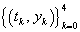. Parameters k1, k2, k3, and k4 are calculated using formulas (4) – (7). tk+1=tk+h (2) yj+1=yj + (k1+2k2+2k3+k4) (3) k1=hf (tj,yj) (4)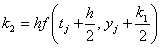(5)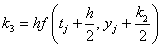(6)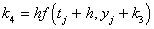(7) Formulas (2) - (7) were implemented in the numerical simulator to solve the nine coupled differential equations in the model. The parameter ranges and initial values in the equations could be specified on the interface of the simulation program. When PSO is used to conduct a search, it is initialized with a group of random particles or solutions and then searches for optimal solutions by updating generations. In every iteration, each particle is updated by following two best values. The first one is the best solution with the best fitness value a particle has achieved so far. The fitness value is also stored. This value is represented by pbest. The second best value that is tracked by the particle swarm optimizer is the best value reached so far by all particles in the population. This best value is a global best and is represented by gbest. When a particle takes part of the population as its topological neighbors, the best value is a local best and is represented by lbest. This results in the local version of PSO. The global version runs faster but might get trapped and potentially converge to a local optimum. Local version runs slower but is not easy to get trapped into a local optimum. In the simulation, the global version was used to get quick results and the local version was used to refine the search. After finding the two best values, the particle updates its velocity and position using the following formulas. v[ ] = v[ ] + c1 * rand( ) * (pbest[ ] - present[ ]) + c2 * rand( ) * (gbest[ ] - present[ ]) (8) present[ ]=persent[ ]+v[ ] (9) Formulas (8) and (9) show the global version of PSO. For the local version, the local best lbest[ ] is used instead of gbest[ ]. v[ ] is the particle velocity. persent[ ] is the current particle position or the solution. pbest[ ] is the individual best. gbest[ ] is the global best. rand( ) is a random number between (0, 1). c1 and c2 are adjustable learning factors. c1=c2 ∈[1.0, 3.0] in our simulation. If the sum of accelerations causes the speed in a dimension to exceed Vmax, which is a parameter specified by the user, the speed in that dimension is set to Vmax. This prevents the particle from possibly moving out an optimal area too fast. The pseudo code of the global version of the PSO technique is as follows. For the local version, lbest is used instead of gbest. For each particle Initialize particle End Do For each particle Calculate fitness value If the fitness value is better than the best fitness value pBest stored set current value as the new pBest End Choose the particle with the best fitness value of all the particles as the gBest For each particle Calculate particle velocity using equation (8) Update particle position using equation (9) Update particle position using equation (9) While predefined maximum number of iterations or minimum error is not reached Since no crossover or mutation operation is involved in the PSO search process, PSO could potentially result in a better performance than genetic algorithms or other evolutional techniques that involve more operations. Simulation Design Figure 1 shows a Graphical User Interface (GUI) that was designed and implemented for the simulation engine so state variable initial values, parameter ranges, and simulation control variables can be specified directly on the interface, which much facilitated the research process using the model. The simulation program can also show the simulation process with current best solutions. The simulator stores all results that meet the criteria in plain text files. Further data mining and graphing can be easily performed on the stored results. A systematic list of test cases was constructed to test the special cases and extreme cases. After each simulation run, the simulation results from the text-based file outputs are obtained and checked against the expected results. For each test case, the generated results should match the expected results. This systematic procedure validates the simulator so it can be used to simulate other cases. Simulation Results The parameters used in the simulation are as follows. Dimension of particles is determined by the number of parameters to be optimized. The simulator counts the number of parameters and uses it as the dimension for particles. The number of particles has a typical range of 20 to 40. For some special scenarios, 100 or 200 particles were tested as well. The particle maximum velocity on each dimension Vmax determines the maximum change a particle can make during any iteration. Vmax can also be specified on the simulator interface. For example, if the particle coordinate ranges are (x1, x2, x3)∈[-10, 10], Vmax is set to 20 for all three dimensions. Different parameter ranges could be specified for different dimensions of particles. After certain dimensions are optimized, their parameter values were fixed to reduce the dimension of the search space and increase the speed of the search process. Figures 2-8 show the comparison between the numerical simulation results and three samples of experimental data for eight variables that have corresponding experimental data . Among them the total tumor volume is a combination of the results from two variables – the MHC class I positive tumor cells volume CMHCI+ and the MHC class I negative tumor cells volume CMHCI−. It was observed that variables were fitted with different error ranges, while the total tumor volume in Figure 6, which is a key state variable, shows a good fit. Considering the varieties of mice tumor data and possibilities of abnormal data items, the trends of all variables were fitted properly in a six-day simulation time frame. Compared to the genetic algorithms, PSO technique resulted in comparable simulation results  using about 60% of the time consumed by genetic algorithms, on average. This shows the performance advantage of the PSO technique over genetic algorithms, besides its simplicity. Search and optimization efficiency is critical for the tumor dynamics models with high-dimensional parameter spaces, which makes PSO a better option when simulating models with similar characteristics. PSO was also analyzed from a methodology point of view. PSO shares many similarities with other evolutionary computation techniques such as genetic algorithms. Both methods start with a randomly generated population, have fitness values to evaluate the population, update the population, and search for the optimum with random techniques. The information sharing mechanism in PSO is significantly different, however. In genetic algorithms, chromosomes share information with each other. The whole population moves like one group towards an optimal area. In PSO, only the best particle gives out the information to others. It is a one-way information sharing mechanism. The evolution only looks for the best solution. Compared with genetic algorithms, all particles tend to converge to the best solution quickly even in the local version in most cases. Unlike genetic algorithms, PSO has no evolution operators such as crossover or mutation. In PSO, particles, or the potential solutions, have memory and update themselves with internal velocities. Compared to genetic algorithms, PSO is easy to implement and there are fewer parameters to adjust in the simulation process, which consumes less simulation resources. The simulation process also shows that some commonly used fitness or goal functions in biological system models such as the sum of error squared and the normalized sum of error squared do not necessarily generate the set of parameters which makes the best fit between model predictions and experimental data. In this project, the data change rate was also considered as a factor and combined with the normalized sum of error squared with adjustable weights in order to result in a better fitness function that evaluates the difference between calculated results and experiment data in a more effective way. The new fitness function resulted in comparable results faster in most cases, although it is not guaranteed since randomness was involved in the search process. Conclusion and Possible Future Work This research project identified PSO as an efficient optimization technique for models with high-dimensional parameter spaces. The simulation results from a tumor cell growth dynamics model showed its simplicity and efficiency. In PSO, a fitness function must be designed to precisely describe how close a given solution is to the optimal in order to facilitate the search process. In the process of modeling biological systems, the selection of fitness functions becomes a challenge due to different scales and large ranges of model variables as well as limited experimental data. A promising future work is to consider more factors in models and develop better fitness functions. Another possible work is to compare the results and search efficiency of PSO with those from other numerical methods such as the Adaptive Markov Chain Monte Carlo (AMCMC) technique, which has been widely used to simulate biological systems. Acknowledgements This study was supported by the National Institute of General Medical Sciences of the National Institutes of Health grant as part of the West Virginia IDeA Network of Biomedical Research Excellence (P20GM103434). References

#### Tables and Figures at a glanceTable 1

#### Figures at a glance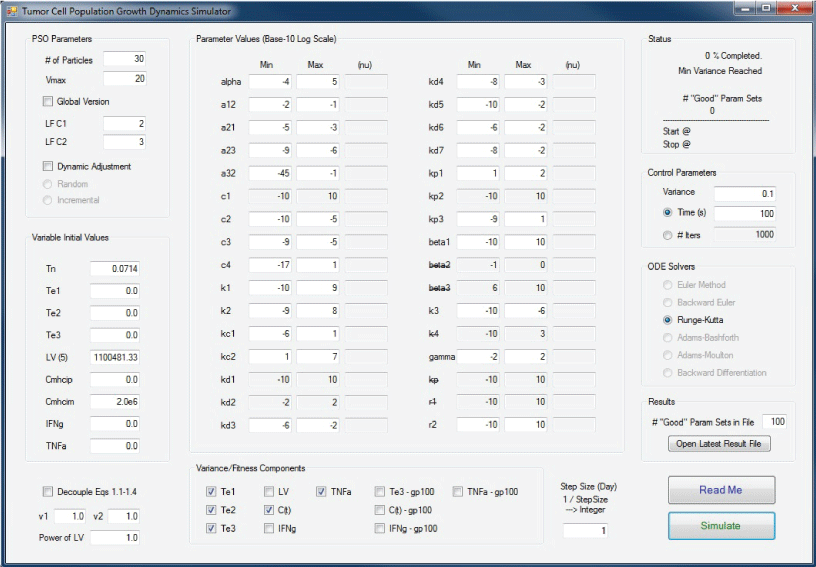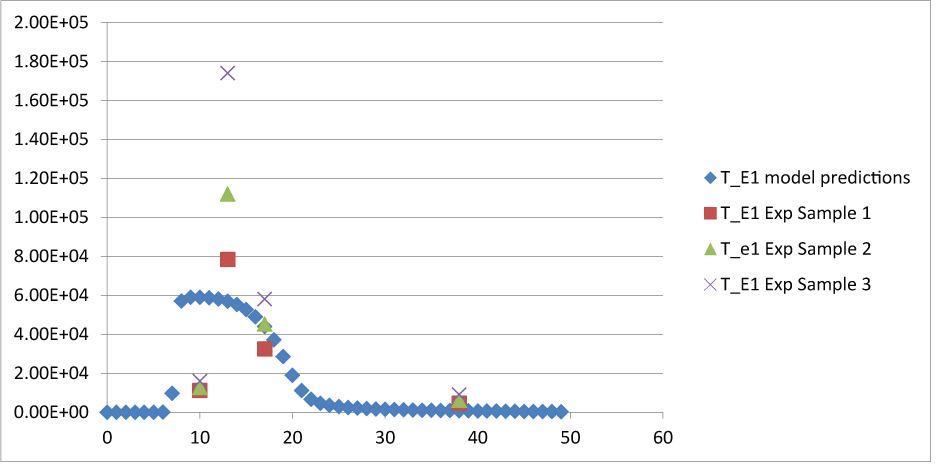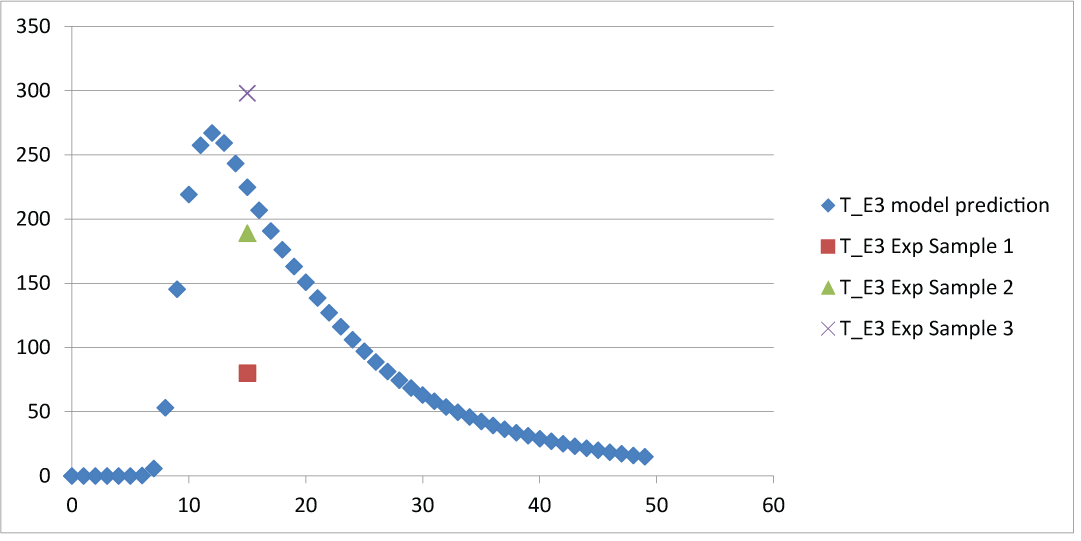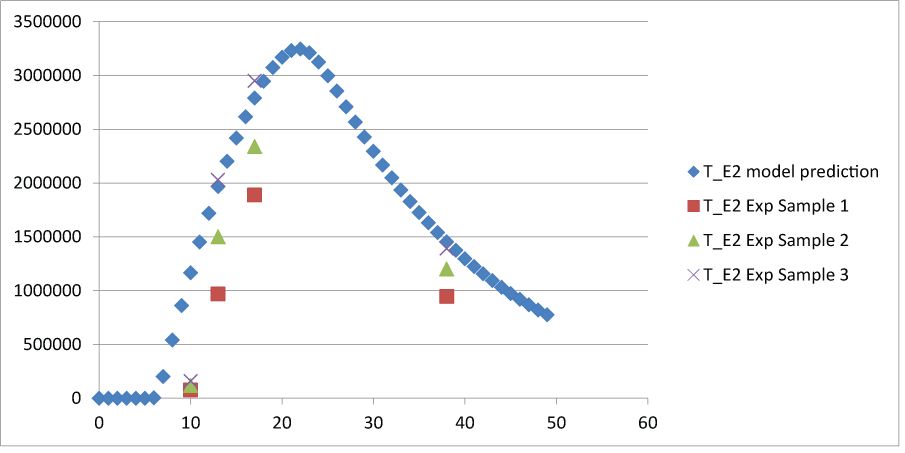Figure 1 Figure 2 Figure 3 Figure 4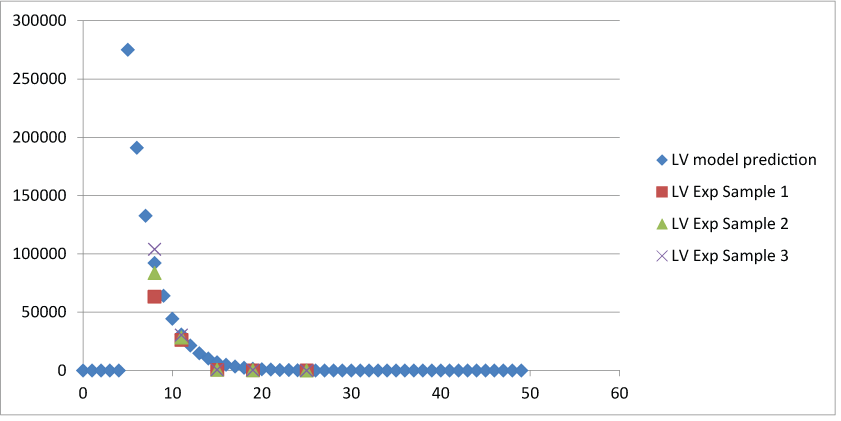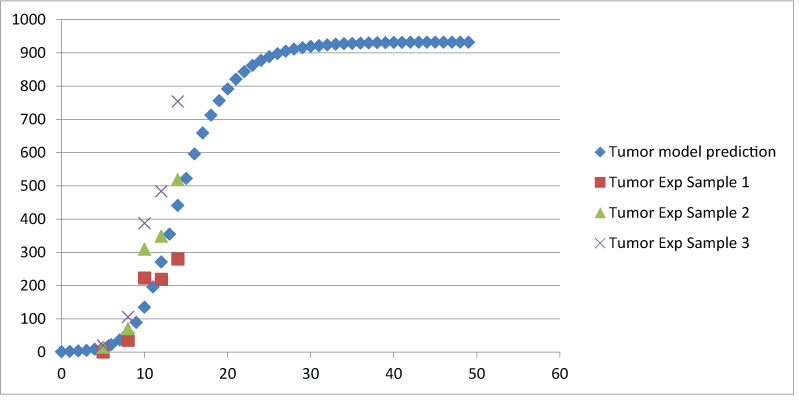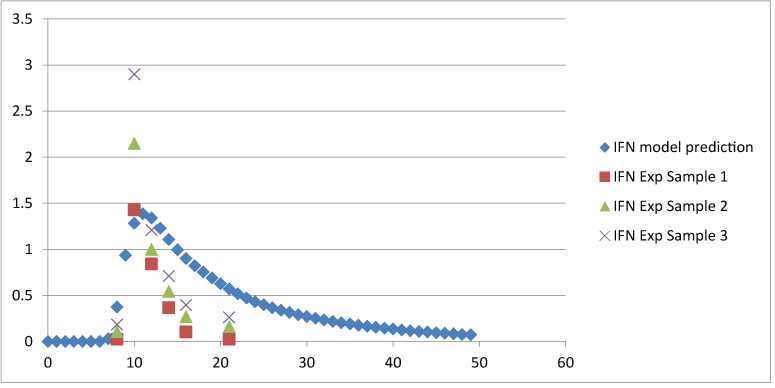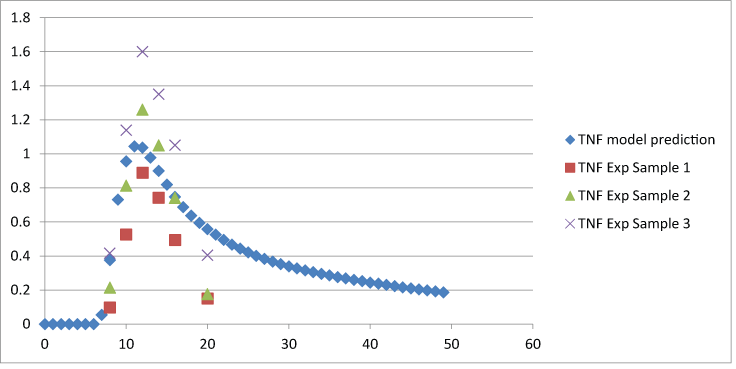Figure 5 Figure 6 Figure 7 Figure 8
Select your language of interest to view the total content in your interested language

### Article Usage

• Total views: 8574
• [From(publication date):
January-2016 - Dec 16, 2019]
• Breakdown by view type
• HTML page views : 8438

## Review summary

1. Jilt
Posted on Sep 21 2016 at 3:45 pm
To address a proposed tumor growth dynamics mathematical model authors method was implemented to speed up the search process in the multi-dimensional parameter space to find optimal parameter values that fit experimental data from mice cancel cells. The manuscript represents the innovative and helpful research for scientific community.Can't read the image? click here to refresh